# Hermite polynomial

In mathematics and physics, Hermite polynomials form a well-known class of orthogonal polynomials. In quantum mechanics they appear as eigenfunctions of the harmonic oscillator and in numerical analysis they play a role in Gauss-Hermite quadrature. The functions are named after the French mathematician Charles Hermite (1822–1901).

• See Addendum for a table of Hermite polynomials through n = 12.

## Orthonormality

The Hermite polynomials Hn(x) are orthogonal in the sense of the following inner product: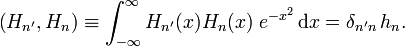$\left(H_{n'}, H_{n}\right)\equiv \int_{-\infty}^\infty H_{n'}(x)H_n(x)\; e^{-x^2}\, \mathrm{d}x = \delta_{n'n}\, h_n.$

That is, the polynomials are defined on the full real axis and have weight w(x) = exp(−x²). Their orthogonality is expressed by the appearance of the Kronecker delta δn'n. The normalization constant is given by$N_n \equiv \sqrt{\frac{1}{h_n}} = \left(\frac{1}{\pi}\right)^{1/4}\, \frac{1}{\sqrt{2^n\,n!}}.$

Normalization is to unity$N_n^2\; \int_{-\infty}^\infty H_{n}(x)H_n(x)\; e^{-x^2}\, \mathrm{d}x = 1.$

The polynomials NnHn(x) are orthonormal, which means that they are orthogonal and normalized to unity.

## Explicit expression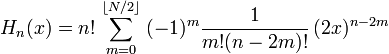$H_n(x) = n!\, \sum_{m=0}^{\lfloor N/2\rfloor} \; (-1)^{m} \frac{1}{m!(n-2m)!} \, (2x)^{n-2m}$

here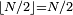$\lfloor N/2\rfloor = N/2$ if N even and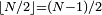$\lfloor N/2\rfloor = (N-1)/2$ if N odd.

## Recursion relation

Orthogonal polynomials can be constructed recursively by means of a Gram-Schmidt orthogonalization procedure. This procedure yields the following relation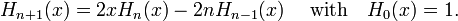$H_{n+1}(x)=2xH_n(x)-2nH_{n-1}(x) \;\quad\hbox{with}\quad H_0(x) = 1.$

The first few follow immediately from this relation,$H_1 = 2x, \quad H_2 = 4x^2 - 2, \quad H_3 = 8x^3 -12x, \quad \ldots$

## Differential equation

The polynomials Hn(x) satisfy the Hermite differential equation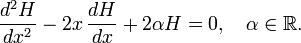$\frac{d^2 H}{dx^2}-2x\,\frac{dH}{dx}+ 2\alpha H=0,\quad \alpha\in\mathbb{R}.$

for the special case$\alpha\in\mathbb{Z}^+$, i.e., for natural positive α.

• If α ≡ n is even, the solution is a polynomial of order n consisting of even powers of x only.
• If α ≡ n is odd, the solution is a polynomial of order n consisting of odd powers of x only.

## Symmetry$H_n(-x) = (-1)^n H_n(x)\;,$

the functions of even n are symmetric under x → −x and those of odd n are antisymmetric under this substitution.

## Rodrigues' formula$H_n(x)= (-1)^n\, e^{x^2}\frac{d^n}{dx^n}\, e^{-x^2}.$

## Generating function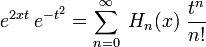$e^{2xt}\,e^{-t^2}=\sum_{n=0}^\infty\;H_n(x)\; \frac{t^n}{n!}$

First few terms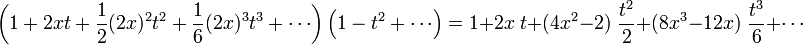$\left(1 +2xt +\frac{1}{2} (2x)^2 t^2 + \frac{1}{6} (2x)^3 t^3+\cdots\right)\left(1 -t^2 +\cdots\right)= 1 + 2x\; t + (4x^2 -2)\;\frac{t^2}{2} + (8 x^3 -12 x)\; \frac{t^3}{6} + \cdots$

so that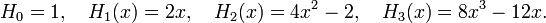$H_0 =1, \quad H_1(x) = 2x,\quad H_2(x) = 4x^2-2, \quad H_3(x) = 8x^3-12x.$

## Differential relation$\frac{dH_n(x)}{dx} = 2n H_{n-1}(x).$

## Sum formula$H_n(x+y) = \left(\frac{1}{\sqrt{2}}\right)^n \sum_{k=0}^n \binom{n}{k} \; H_k(\sqrt{2}\,x) \; H_{n-k}(\sqrt{2}\,y),$

where$\binom{n}{k}$ is a binomial coefficient.